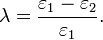# Lambda Coefficient: Simple Definition

Goodman and Kruskal’s lambda coefficient measures the proportional reduction in error in crosstab analysis. The statistic can be symmetric, where you do not have to specify which variable is dependent, and asymmetric where the dependent variable is specified.ε1 is the overall non-modal frequency and ε2 is the sum of the non-modal frequencies for each value of the independent variable.

## Use of the Lambda Coefficient

The lambda coefficient isn’t used very often, perhaps because the underlying calculations are “…not generally understood by behavioral scientists” (Hartwig, 1973). Despite some “major weaknesses” (source), it is useful as a way to understand how variables relate to each other. Specifically, λ shows how one variable’s values can help to reduce prediction errors in a second variable.

## References

Hartwig, F.: Statistical significance of the lambda coefficients. Behavioral Science 18 (1973), 307-310

CITE THIS AS:
Stephanie Glen. "Lambda Coefficient: Simple Definition" From StatisticsHowTo.com: Elementary Statistics for the rest of us! https://www.statisticshowto.com/lambda-coefficient/
---------------------------------------------------------------------------Need help with a homework or test question? With Chegg Study, you can get step-by-step solutions to your questions from an expert in the field. Your first 30 minutes with a Chegg tutor is free!